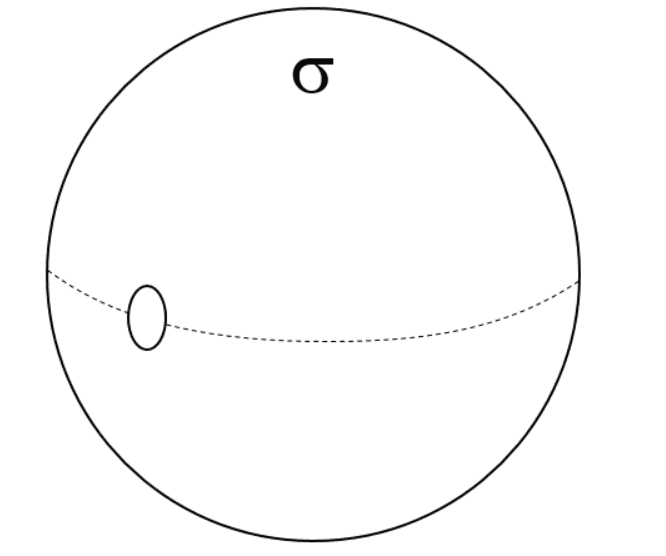# Gauss' Law applied to this Charged Spherical Shell with a small hole

• Jaccobtw
In order to use a gaussian surface like this you must satisfy several conditions. In particular, your surface must use a symmetry of the problem to be able to conclude what the flux through the surface is in terms of the field, which must be orthogonal to the surface based on the symmetry (or parallel to the surface in parts of the surface where you want zero flux). This is not the case here.In summary, a spherical shell of uniform charge has a circular hole cut out of it. The Electric Field at a radius just outside the sphere is $\sigma R^2$.f

#### Jaccobtw

Homework Statement
A spherical shell of uniform charge density σ has a circular hole cut out of it as shown below. What is the Electric Field at a radius just outside the sphere, directly over the center of the circular, cut-out hole? HINT: the hole is small enough that you can treat it as flat, and the point at which you are calculating the field is so close to the hole that it can be approximated as an infinite plane.
Relevant Equations
$$\oint_{}^{} E \cdot dA = \frac{q_e}{\epsilon_o}$$First draw a gaussian shape outside of the sphere (a larger sphere) with radius R. The total charge from the (inner) sphere will be:

$$Q = \sigma A$$
$$A = 4\pi r^2$$
$$Q = \sigma 4\pi r^2$$

Use Gauss's Law to derive electric field magnitude

$$\oint_{}^{} E \cdot dA = \frac{q_e}{\epsilon_o}$$
$$E\oint_{}^{} dA = \frac{q_e}{\epsilon_o}$$
$$EA = \frac{q_e}{\epsilon_o}$$
$$E = \frac{q_e}{\epsilon_oA}$$

Substitute for q and A

$$E = \frac{\sigma4\pi r^2}{\epsilon_o4\pi R^2}$$

Cancel

$$E = \frac{\sigma r^2}{\epsilon_o R^2}$$

I'm not surprised that this is wrong, but I feel like I should be allowed to use more variables. Anyways, can anyone help me to see what I did incorrectly here?

Much appreciated

Last edited by a moderator:
You have computed just the field from a sphere with constant surface charge, not solved the actual problem (which was a sphere with a circular patch removed).

You have computed just the field from a sphere with constant surface charge, not solved the actual problem (which was a sphere with a circular patch removed).
Is the small patch removed dA?

Last edited:
You have computed just the field from a sphere with constant surface charge, not solved the actual problem (which was a sphere with a circular patch removed).
Is there enough information from the question to answer it?

Is there enough information from the question to answer it?
Yes.

Homework Statement:: A spherical shell of uniform charge density σ has a circular hole cut out of it as shown below. What is the Electric Field at a radius just outside the sphere, directly over the center of the circular, cut-out hole? HINT: the hole is small enough that you can treat it as flat, and the point at which you are calculating the field is so close to the hole that it can be approximated as an infinite plane.
Relevant Equations:: $$\oint_{}^{} E \cdot dA = \frac{q_e}{\epsilon_o}$$

View attachment 298551
...
$$E = \frac{\sigma4\pi r^2}{\epsilon_o4\pi R^2}$$
Cancel
$$E = \frac{\sigma r^2}{\epsilon_o R^2}$$

I'm not surprised that this is wrong, but I feel like I should be allowed to use more variables. Anyways, can anyone help me to see what I did incorrectly here?

Much appreciated
Hint: Use the principle of superposition, the hole having surface charge density of ##-\sigma##, and a sphere with no hole.

•PhDeezNutz
Well you can use symmetry because the hole is small enough. The piece of area opposite the hole has an electric field pointing out I think
Hint: Use the principle of superposition, the hole having surface charge density of ##-\sigma##, and a sphere with no hole.
how does the hole have a surface charge density of -##\sigma##?

how does the hole have a surface charge density of -σ?
The hole has no charge. Therefore, to get to that situation from a uniformly charged sphere with charge density ##\sigma## you must subtract a charge density ##-\sigma## where the hole is.

The hole has no charge. Therefore, to get to that situation from a uniformly charged sphere with charge density ##\sigma## you must subtract a charge density ##-\sigma## where the hole is.
What if you use a cylinder to enclose the empty hole and the other side of the sphere. If you do that I think you get ##\sigma / 2 \epsilon_o ##

What if you use a cylinder to enclose the empty hole and the other side of the sphere. If you do that I think you get ##\sigma / 2 \epsilon_o ##
That's an incorrect method.

That would imply the the E-field Just outside the sphere of the side opposite this very small hole also has magnitude ##\dfrac{\sigma }{ 2 \epsilon_0 } ##: half what the field is nearby.

No way !

What if you use a cylinder to enclose the empty hole and the other side of the sphere. If you do that I think you get ##\sigma / 2 \epsilon_o ##
In order to use a gaussian surface like this you must satisfy several conditions. In particular, your surface must use a symmetry of the problem to be able to conclude what the flux through the surface is in terms of the field, which must be orthogonal to the surface based on the symmetry (or parallel to the surface in parts of the surface where you want zero flux). This is not the case here.

You want to use the hint of the problem to consider the hole as an infinite sheet.

•SammyS